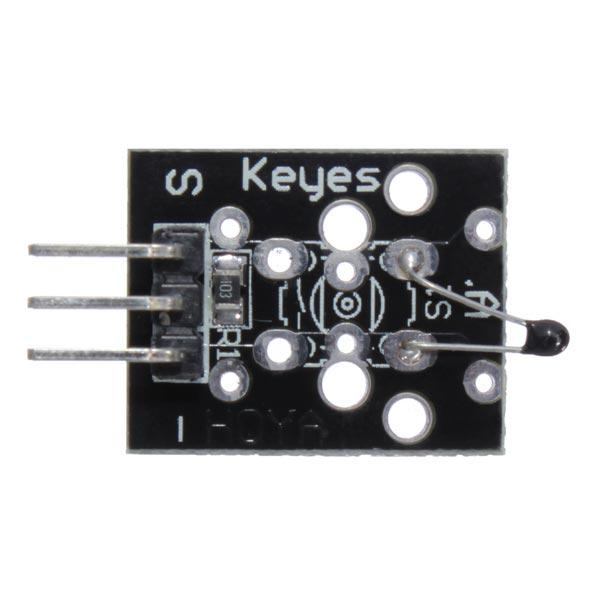# KY-013 Analog Temperature Sensor Module

8,198

The KY-013 Analog Temperature Sensor Module uses a thermistor to measure ambient temperature based on the resistance of the device.• The temperature sensor is a NTC thermistor
• Multi-point temperature measurement Measures temperatures: -55°C / +125°C
• Accuracy: + / – 0.5°C

Connection

Connect board’s power line (middle) and ground (-) to 5V and GND respectively. Connect signal (S) to pin A0 of the Arduino.

 KY-013 Arduino S A0 middle 5V – GND

Code

 Source code```#include <math.h>

double Temp;
Temp = 1 / (0.001129148 + (0.000234125 + (0.0000000876741 * Temp * Temp ))* Temp );
Temp = Temp - 273.15; // Convert Kelvin to Celcius
return Temp;
}

void setup() {
Serial.begin(9600);
}

void loop() {